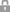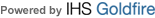### Products & Services

• Description: The Model VD-301 capacitive voltage divider is intended for the measurement of voltage amplitude and wave-shape of ac signals at high potential. It has a nominal division ratio of 5000:1, and the exact measured ratio is printed on the name-plate. This ratio is measured in

• Form / Technology: Voltage Divider
• Output Voltage Range: 400000 volts

• Description: Pearson high voltage coaxial capacitive voltage dividers permit measurements up to 500kV when used in high voltage insulating oil. Customized division ratio and calibration for use in air are available. See the table below for model specifications.

• Form / Technology: Voltage Divider
• Output Voltage Range: 300000 volts

• Description: The Model VD-305A capacitive voltage divider is intended for the measurement of voltage amplitude and wave-shape of ac signals at high potential. It has a nominal division ratio of 5000:1, and the exact measured ratio is printed on the name-plate. This ratio is measured in air

• Form / Technology: Voltage Divider
• Output Voltage Range: 50000 volts

• Description: The Model VD-500A capacitive voltage divider is intended for the measurement of voltage amplitude and wave-shape of ac signals at high potential. It has a nominal division ratio of 10000:1, and the exact measured ratio is printed on the name-plate. This ratio is measured in

• Form / Technology: Voltage Divider
• Output Voltage Range: 500000 volts

### Find Suppliers by Category TopIndicates content that may require registration and/or purchase.